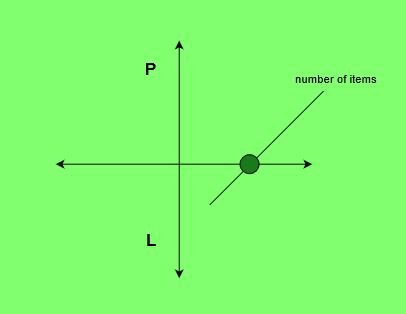Related Articles

# Program to find the Break Even Point

• Last Updated : 15 Apr, 2021

Given the list of monthly expenditureof an organization, selling priceof each item, the task is to calculate the Break Even Point.
Break Even Point refers to the number of items sold in order to neutralize the total expenditure i.e. Overall, neither profit nor loss.
Examples:

Input: Expenditure = 18000, S = 600, M = 100
Output: 36
We need to sell 36 items to cover expenditure and maintenance overhead
Input: Expenditure = 3550, S = 90, M = 65
Output: 142Approach:

1. Calculate the sum of all the expenditures.
2. Subtract the maintenance (Cost price) from the selling price.
3. Divide the expenditure sum by the above-obtained amount to get the minimum number of items to be sold (Break Even Point).

Below is the implementation of the above approach:

## C++

 // C++ program to find the break-even point. #include #include using namespace std; // Function to calculate Break Even Pointint breakEvenPoint(int exp, int S, int M){    float earn = S - M;     // Calculating number of articles to be sold    int res = ceil(exp / earn);     return res;} // Main Functionint main(){    int exp = 3550, S = 90, M = 65;    cout << breakEvenPoint(exp, S, M);    return 0;}

## Java

 // Java program to find Break Even Pointimport java.io.*;import java.lang.*; class GFG{// Function to calculate// Break Even Pointpublic static int breakEvenPoint(int exp1,                                 int S, int M){    double earn = S - M;         double exp = exp1;     // Calculating number of    // articles to be sold    double res = Math.ceil(exp / earn);     int res1 = (int) res;         return res1;} // Driver Codepublic static void main (String[] args){    int exp = 3550, S = 90, M = 65;    System.out.println(breakEvenPoint(exp, S, M));}} // This code is contributed// by Naman_Garg

## Python 3

 # Python 3 program to find# Break Even Pointimport math # Function to calculate# Break Even Pointdef breakEvenPoint(exp, S, M):         earn = S - M     # Calculating number of    # articles to be sold    if res != 0:      res = math.ceil(exp / earn)    # if profit is 0, it will never make ends meet    else:      res = float('inf')             return res     # Driver Codeif __name__ == "__main__" :             exp = 3550    S = 90    M = 65         print (int(breakEvenPoint(exp, S, M))) # This code is contributed# by Naman_Garg

## C#

 // C# program to find Break Even Pointusing System; class GFG{// Function to calculate// Break Even Pointpublic static int breakEvenPoint(int exp1,                                int S, int M){    double earn = S - M;         double exp = exp1;     // Calculating number of    // articles to be sold    double res = Math.Ceiling(exp / earn);     int res1 = (int) res;         return res1;} // Driver Codepublic static void Main (){    int exp = 3550, S = 90, M = 65;    Console.WriteLine(breakEvenPoint(exp, S, M));}} // This code is contributed// by inder_verma..

## PHP

 

## Javascript

 
Output:
142

Attention reader! Don’t stop learning now. Participate in the Scholorship Test for First-Step-to-DSA Course for Class 9 to 12 students.

My Personal Notes arrow_drop_up